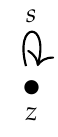>**Puzzle 107.** Take the free category on this graph:

>>and then impose the equation \$$s \circ s \circ s \circ s = 1_z\$$. You get a category with one object, also known as a **[monoid](https://en.wikipedia.org/wiki/Monoid)**. How many morphisms does this category have? How is it related to the picture below?

>To simplify the proof, let,
\$s^{\circ n}=\underbrace{s\circ s\circ s\circ\cdots s}\_{n \text{ times}} \\\\ \\#\lbrace s^{\circ n} \rbrace= \\#\lbrace f \mid f=s^{\circ n}\rbrace \$

When \$$n=1\$$,
\$s^{\circ 1}=s \\\\ \\#\lbrace s^{\circ 1} \rbrace= 1. \$
When \$$n=2\$$,
\$s^{\circ 2}= s\circ s \\\\ \\#\lbrace s^{\circ 2} \rbrace= 1. \$

When \$$n=3\$$,
\$s^{\circ 3}=s\circ s\circ s \\\\ \\#\lbrace s^{\circ 3} \rbrace= 1. \$

When \$$n=4\$$,
\$s^{\circ 4}=s\circ s\circ s\circ s=1_z \\\\ \\#\lbrace s^{\circ 4} \rbrace= 0. \$
Summing up all the terms,
\$3=\sum_{n=1}^{4}\\#\lbrace s^{\circ n} \rbrace \$
Giving an answer of \$$3\$$.

Edit: Are we also counting \$$1_z\$$? Otherwise, this gives a different answer.